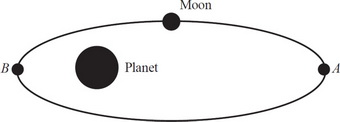# AP Physics 1 Question 65: Answer and Explanation

### Test Information

Question: 65

5.A moon has an elliptical orbit about the planet as shown above. At point A, the moon has speed vA and is at a distance rA from the planet. At point B, the moon has a speed of vB. Which of the following correctly explains the method for determining the distance of the moon from the planet at point B in the given quantities?

• A. Conservation of angular momentum, because the gravitational force exerted by the moon on the planet is the same as that exerted by the planet on the moon
• B. Conservation of angular momentum, because the gravitational force exerted on the moon is always directed toward the planet
• C. Conservation of energy, because the gravitational force exerted on the moon is always directed toward the planet
• D. Conservation of energy, because the gravitational force exerted by the moon on the planet is the same as that exerted by the planet on the moon